# Engineering Thermodynamics: Fundamentals & Principles

The fundamentals of engineering thermodynamics play an important role in moving towards a better world, through improvement in performance of the plant, equipment, and their overall design.

Factors that are critical in assessing the performance of the equipment are items like the output of the final product, consumption of input raw material, production cost, and assessment of the effect on environmental. Engineers today are using the concept of thermodynamics to examine and reinvent things which are intended for human safety and comfort.

The science of thermodynamics has existed since the 19th century. Since then scientists and engineers have been making a constant and continuous effort of making it as user-friendly as possible.

## Fundamentals of Thermodynamics

The word thermodynamics is derived from the Greek word theme (means heat) and dynamics (means force). Engineering professionals are interested in studying systems and their interaction with their surroundings.

Concepts/Definitions used in this section are helpful for readers in understanding the concept of engineering thermodynamics (sometimes referred to as Heat-Power Engineering)

### System, Surrounding and Universe

A system is something which we want to study and interested in, thus the first step is to fix precisely the objective of system study. The objective system study can be improving the efficiency of the system or to reduce the losses etc. Example of System can be to analyze the refrigeration cycle in cold storage plant or to analyze the Rankine cycle in a power plant.

A system is defined as a definite mass of pure substance bounded by a closed or flexible surface; similarly, the composition of matter inside the system can be fixed or variable depending upon the cycle.

System dimensions are not necessarily constant (like air in a compressor is compressed by a piston) it can be variable (like an inflated balloon). The matter which interacts with the system externally is called Surrounding and the Universe is the outcome of system and surrounding.

The element which separates the system from its surrounding is called boundary. The boundary of the system can be fixed or in motion.

The interaction between the system and the surrounding takes place by crossing the boundary and thus plays a very important role in thermodynamics (i.e. heat and power engineering).

### Types of System in Thermodynamics

There are two basic types of systems in thermodynamics:

1. Closed System or Control Mass: is associated with the definite quantity of man atter. Unlike an open system, in a closed system, there is no mass flow of matter occurs across the boundary of the system. There is also a special type of closed system which does not interact and isolated itself from the surrounding is called an isolated system.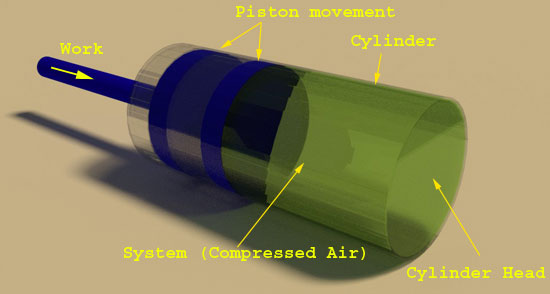2. Control Volume (Open System): Control volume is limited to a region of space through which mass and energy can flow and cross the boundary of the system. The boundary of an open system is called a controlled surface; this controlled surface can be actual or unreal.
Examples of control volume are types of equipment that involve the flow of mass to cross the boundary of the system such as the flow of water through pumps, steam flow in turbines and air flow through air compressors.

## Microscopic Thermodynamics

The microscopic approach in thermodynamics is also called statistical thermodynamics and is associated with the structure of matter and the objective of the statistical thermodynamics is to characterize the average behavior of the particle making up the system of interest and in turn, used this information to observe the macroscopic behavior of the system.

## Thermodynamics Property, States and Process

### Thermodynamic Property

A thermodynamic property is a macroscopic characteristic of a system. The value of a property can be assigned at any given time without the knowledge of previous value and its behavior.

### Extensive Property

Properties that are dependent on mass are called extensive properties and its value for the overall system is the summation of its values for the parts into which the system is divided. Examples of extensive property are Volume, Energy, and Mass. Extensive property depends upon the size of a system and it can change with time.

### Intensive Property

In contrast to the extensive property, an intensive property is not mass dependent and non additive in nature and does not depend upon on the total size of the system. It can vary at different places within the system at any moment. Examples of intensive property are pressure and temperature.

### Thermodynamic State

A state is defined as the condition of a system which is best described by its properties. The mass enclosed in a system can be found in a variety of the unique conditions, called state. There are relations among the properties of a system but the state can be specified by providing the value of a subset of the properties.

### Thermodynamic Process

Thermodynamic processes are the conversion of one state to another state. If the value of the macroscopic property of the in a system at two different time are identical then the system is said to be in a same state at that time. Steady state condition of the system is achieved if none of its properties changes with respect to time.

## System Equilibrium Cycle

A thermodynamic system equilibrium cycle is a sequential process that starts and terminates with the condition of the same state. When the cycle completes then it’s all properties are having the same value what they were at the beginning. All cycles that repeats regularly plays a vital role in many areas of application, like the circulation of condensate in a thermal power generating station executes a cycle.

## Working Substance

Theory of matter is helpful in understanding the concept of energy. Matter is known for its mass, volume and space and irrespective of its structure and nature it has certain characteristics like consistency and reliability. Matter is made from a large number of particles called molecules. One can find matters of Solid, liquid or gas everywhere.

In solid matter, molecules are close to each other and strongly bounded and cannot able to move freely. Thus large force required to change its shape.

Molecules in a liquid matter are not firmly held and thus a very small force is sufficient to keep the molecules together.

In a gaseous state the molecules moves randomly and freely as if it is in an unbound state then it moves very fast irrespective of its adjacent molecules. Compressibility is associated with gases, are having plenty of empty spaces between the connecting molecules. Energy is the reason for the matter to exist in different phases.

## Pure Substance

Material of solo chemical structure or homogeneity in variant chemical structure is known as pure substances. Material can exist in a single phase like liquid or can also exist in more than one phase in equilibrium with each other. A uniform mixture of gases having similar chemical composition is also termed as a pure substance.

The importance of pure substance is in the determination of properties of the working substance at different conditions of pressure and temperature.

Example: For pure substance like water can be described fully by two sovereign intensive properties termed as pressure and temperature. Another pure substance is air in the gaseous state. But for non homogenous substance, more than two properties are required to describe the state.

## Thermodynamic Equilibrium

In mechanics, equilibrium is said to have reached when we equalises the opposing forces. But the meaning of thermodynamic equilibrium is different and far reaching as it involves balancing act for many other influences (between and system and surrounding) apart from balancing opposing forces). In order to attain the complete equilibrium with in a system, one need to fulfil the condition for mechanical, thermal, phase and chemical equilibrium.

In this section, we are limiting our discussion to thermodynamic equilibrium. Emphasis on having equilibrium states and its change from one equilibrium to another is best described by Classical Thermodynamics.

If the state is fixed then the system is said to be in equilibrium. Intensive properties like pressure and temperature to be accurately measured in order to assign the state. A system is said to be in thermodynamic equilibrium if its intensive properties not changes on account of very little disturbance.

Under this situation, the system is in complete stability with the restraints offered by the surroundings.

## Actual and Quasi-equilibrium Process

During an actual process, a system can be considered as non-equilibrium due to the various non equilibrium effects present in the system and thus shows a change in pressure and temperature.

In the Quasi-equilibrium (quasi-static) process the deviation from the thermodynamic equilibrium state is extremely small. Thus all states through which the system passes is considered to be in equilibrium state during a Quasi-equilibrium process.

Quasi-equilibrium is used for deriving relation-ships among various extensive properties like entropy, internal energy, specific heats and enthalpy etc.

## Dimensions and Units

Physical quantity is known by its dimensions and if the magnitude is given to this dimension then it is termed as a unit. Prerequisite of engineering calculations is to have the same unit of physical quantities.

Some vital dimensions like mass (m), length (L), time (t), and temperature (T) are termed as primary-dimensions. Engineers and scientists around the globe are performing their calculations mainly on two types of units called the English system and Metric system (SI). SI system of unit representation is more logical and commonly used by professionals.

 Primary Dimensions Metric (SI) Unit English Unit Length Meters (m) Foot(ft) Mass Kilogram (kg) Pound (lb) Time Seconds (s) Seconds (s) Temperature Kelvin (K) Fahrenheit Electric Current amperes (A) amperes (A)

## Secondary Dimensions and Units

Secondary dimensions or derived dimensions are expressed in terms of primary dimensions like Velocity (V), Energy (E), and Volume (V), Force, Power, Heat etc.

Force is considered as a secondary dimension in SI units, since its unit is derived from Newton’s second law that is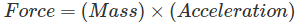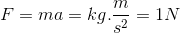Force is defined as the force required to accelerate a mass of 1 kg at a rate of 1 m/s2.
Weight and mass are not to be considered as same. Weight is gravitational-force act on a body and its magnitude is determined from Newton’s second law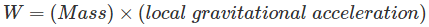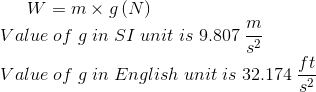Specific Weight(y) is defined as the gravity force acting on a unit volume of a substance and is determined by y = (density ) × g N/ m3
Thus regardless of location in the universe, the mass of the body remains the same. When gravitational acceleration changes then the weight of the body also changes. At top of the mountain, the body weighs less, as its g decreases with altitude.

Specific Volume(ϑ) and Density(ρ) both are intensive property and can differ from point to point. Reciprocal of density is a specific volume.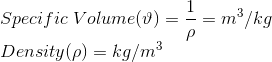Pressure: Pressure is defined as a normal force exerted by a fluid per unit area in case of liquid or gas. In solid pressure is equivalent to normal stress.

SI Unit of pressure and stress is Pascal (N/ m2). Other units of pressure are given below:

 1 Pascal 1 N/ m2 1 K – Pascal 103 – N/ m2 1 bar 105 – N / m2 1 M – Pascal 106 – N/ m2 1 atm 101.325 kpa=1.01325 bars 1 Bar 100 kpa = 0.1 M – Pascal 1 kgf/cm2 9.807 N/cm2 = 0.9807 Bar = 0.9679 atm

Absolute pressure is related with the actual pressure at a given point and the measurement is done with respect to absolute vacuum or absolute zero pressure.
The actual pressure at a given point is refered as absolute pressure and is measured relative to absolute vacuum (absolute zero pressure).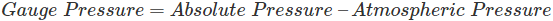Pressures below atmospheris pressure are called Vacuum pressure and measured by vacuum gauge that indicates the difference between ‘atmospheric pressure and the ‘absolute pressure.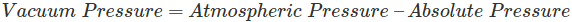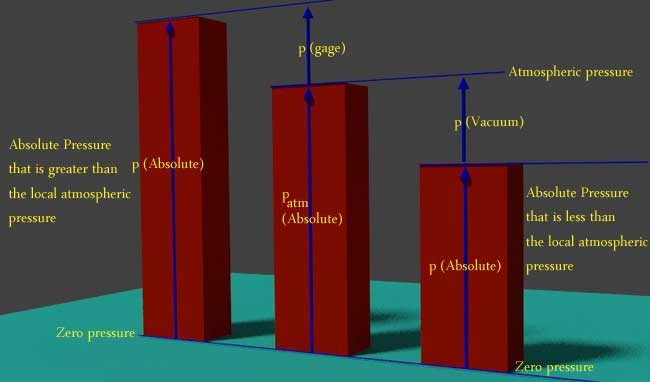The above figure best describe the relationship between absolute pressure, atmospheric pressure, gauge pressure and vacuum pressures

## Energy and forms of Energy

Energy is defined as the capability to do work. Energy input always produces some effect on the matter of the system.

Two-major types of energy are: Stored Energy and Energy in Transit

1. In material stored energy can exit in several forms like Internal Energy (IE), Kinetic energy (KE), Potential Energy (PE), Chemical Energy (CE), Electrical Energy (EE), Nuclear Energy (NE). Details of this energy will be discussed in the next section.
2. Energy in Transit

Heat
Heat is defined as the energy transferred without the transfer of mass across the boundary of a system due to the temperature difference between the system and the surroundings. The energy in transition alone is called heat. The amount of heat transferred during a process is dependent on the path followed and not on the end conditions only.

WorkWhen force F is used to move one or more particle through a distance x then it is called Work. In a given below piston cylinder arrangement in order to decrease volume V of a system, work is required to be done. Therefore in an extremely small volume change in the system as a result of the motion of the piston is related to the differential in work through the force-distance product by the formula:

dW = Fdx = pAdx = pdV [ ft-ldf | Nm] …………….. (1)
dw = pdv [Btu / lbm | KJ/kg ] …….. (2)
Where, p is system-pressure, A is the area, F is the force, x is Incremental distance-travelled, W is work, V is volume

Lower case letters in equation 1 and 2 above are for work and volume based on per-unit mass. Extensive properties with lower case characters are called specific-properties.
Equation- (1) is in English unit, while British thermal unit (BTU) is used in Equation- 2 and the two sets of units are linked by a conversion factor called Mechanical-Equivalent of heat and its value is 778 ft-lbf / Btu.

Want To Learn Faster? 🎓
Get electrical articles delivered to your inbox every week.
No credit card required—it’s 100% free.